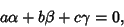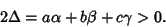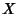## Line at Infinity

The straight line on which all Points at Infinity lie. The line at infinity is given in terms of Trilinear Coordinates bywhich follows from the fact that a Real Triangle will have Positive Area, and therefore thatInstead of the three reflected segments concurring for the Isogonal Conjugate of a pointon the Circumcircle of a Triangle, they become parallel (and can be considered to meet at infinity). Asvaries around the Circumcircle,varies through a line called the line at infinity. Every line is Perpendicular to the line at infinity.